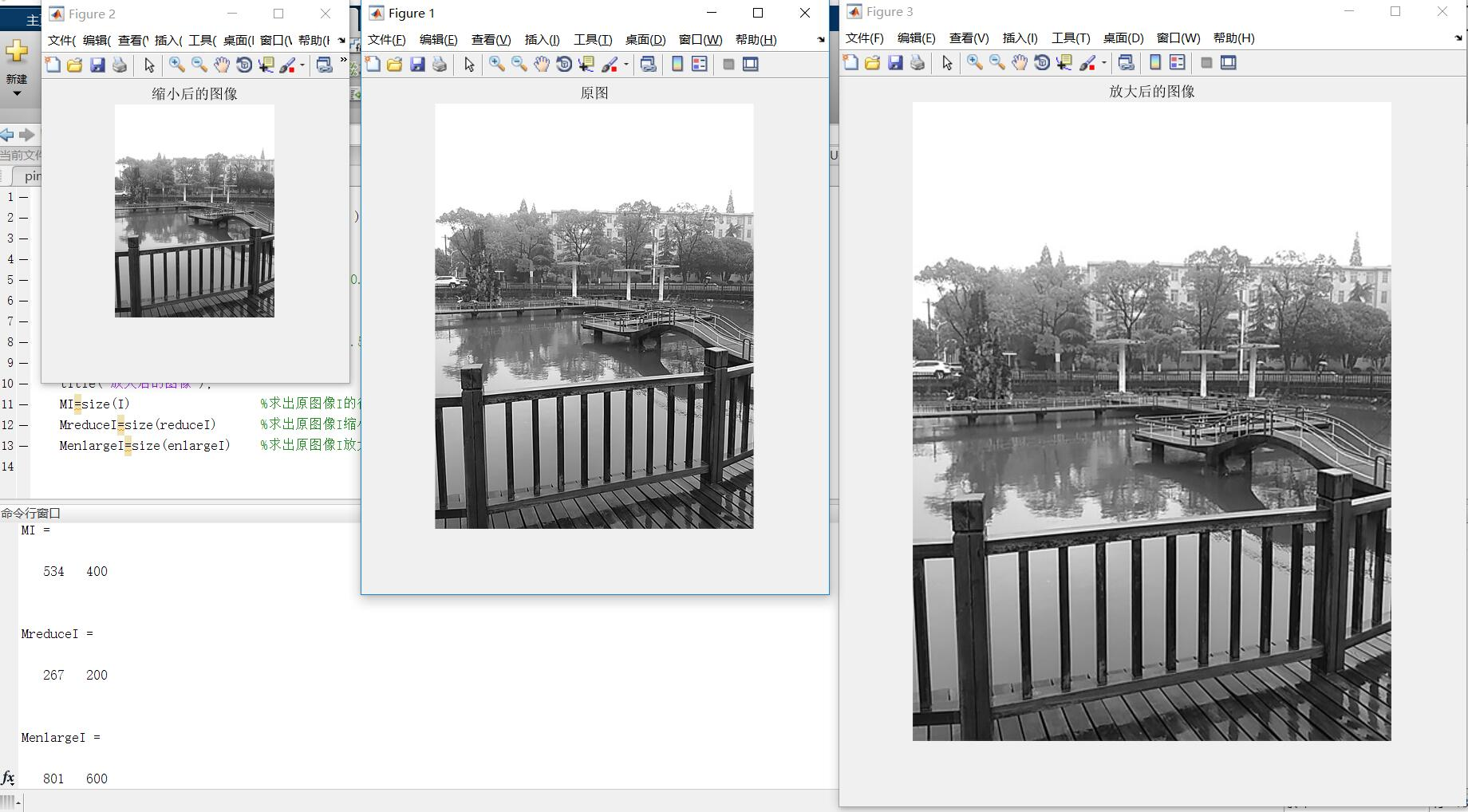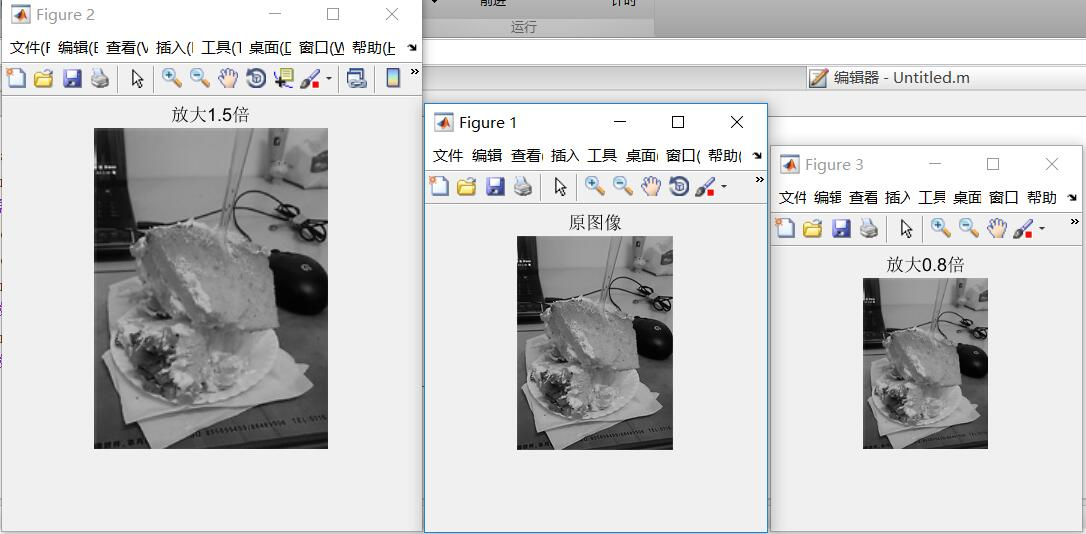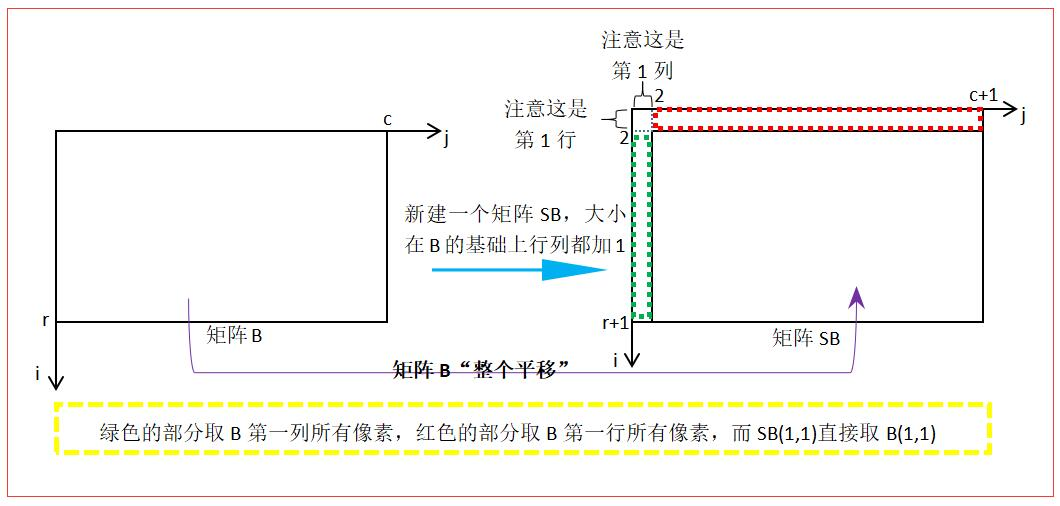• matlab图像缩放
千次阅读
2018-10-21 17:31:33

要求：
将指定文件夹中的所有图片一次缩小后保存，名字与原来的名字相同。
核心语句：

S=imresize(rgb,1/6,'nearest');


整体代码：

file_path =  'F:\2018\';   % 图像文件夹路径
img_path_list = dir(strcat(file_path,'*.jpg'));   %获取该文件夹中所有jpg格式的图像
img_num = length(img_path_list);   %获取图像总数量
if img_num > 0    %有满足条件的图像
for j = 1:img_num    %逐一读取图像
image_name = img_path_list(j).name;  % 图像名
S=imresize(rgb,1/6,'nearest');   %缩小六分之一
imwrite(S,image_name);    %将缩小后的图片保存
end
end

更多相关内容
• 目录一、插值与图像缩放二、最近邻插值1、原理2、代码实现三、双线性插值1、原理2、代码实现 一、插值与图像缩放   首先举个例子说明插值过程，先看看matlab的插值函数 interp() 吧： x = -2 : 1 : 2; y = -2 : 1 ...
• 图像缩放，是用matlab实现的子函数，简单快捷。能够缩放任意尺寸。
• Matlab实现的对图像双线性插值进行缩放，里面包含两次缩放
• 使用临近插值法和双线性插值法进行图像处理，将彩色图像缩放之后仍输出彩色图像
• matlab图像处理实例，包含了图像变换，剪切，缩放，滤波等各方面实例。
• matlab实现对图像的双三次卷积内插，以图像缩放为例子
• MATLAB图像缩放,matlab实现图像缩放,matlab源码
• 包含最近邻插值、双线性插值和三次插值的matlab代码，自己已经测试过，使用的时候注意将图片转换为位图，否则会出现错误。
• 运用matlab程序，给定缩放比，程序自动缩放图片大小
• 自己用MATLAB制作的图片浏览器，目前只能实现图片的打开、向前/向后翻页、图片缩放等功能。
• matlab实现图像缩放 缩放同样是仿射变换的一种特例，它接受水平和垂直两个方向的缩放值。 matlab 实现水平，垂直，水平垂直缩放： clear all close all clc img = imread('test.jpg'); %读取输入图片的...

# matlab实现图像的缩放

缩放同样是仿射变换的一种特例，它接受水平和垂直两个方向的缩放值。matlab 实现水平，垂直，水平垂直缩放：

clear all
close all
clc
[h,w,d] = size(img); %获取行和列，即原图的高度和宽度
xscale = 3;
yscale = 3;
ht = floor(h./xscale);
wt = floor(w./yscale);
%zoo = zeros(ht,w,d);
for i = 1:ht
for j = 1:w
% for k = 1:d
%zoo(i,j,d) = img(floor(i*xscale),j,d);
% end
zooh(i,j) = img(floor(i*xscale),j);
end
end

for i = 1:h
for j = 1:wt
zoow(i,j) = img(i,floor(j*xscale));
end
end

for i = 1:ht
for j = 1:wt
zoohw(i,j) 
展开全文• Matlab图像缩放 Matlab实现函数代码如下： clc I=rgb2gray(imread('Campus-scenery2sk.JPG')); figure,imshow(I); title('原图'); reduceI=imresize(I,0.5); %原图像I缩小0.5倍 figure,imshow(reduceI); title('...

Matlab图像缩放

Matlab实现函数代码如下：

clc
figure,imshow(I);
title('原图');
reduceI=imresize(I,0.5);     %原图像I缩小0.5倍
figure,imshow(reduceI);
title('缩小后的图像');
enlargeI=imresize(I,1.5);   %原图像I放大1.5倍
figure,imshow(enlargeI);
title('放大后的图像');
MI=size(I)                  %求出原图像I的行列并在命令窗口显示
MreduceI=size(reduceI)      %求出原图像I缩小后的图像的行列并在命令窗口显示
MenlargeI=size(enlargeI)    %求出原图像I放大后的图像的行列并在命令窗口显示


函数运行的结果如下图所示：
-程序中的函数说明：
B=imresize(A,scale,method);
A为要进行缩放的图片，scale为缩放的倍数，可选项method为缩放时采用的插值方法，默认值为最近邻插值。
设( x0,y0 $x_0,y_0$)为原图像上的一点，我们对其进行缩放处理，设x方向的缩放倍数为 Sx $S_x$,设y方向的缩放倍数为 Sy $S_y$, (x1,y1) $(x_1,y_1)$为缩放后的像素点坐标，用矩阵变换表示为：
[x1y11] $\begin{bmatrix}x_1&y_1&1\end{bmatrix}$= [x0y01] $\begin{bmatrix}x_0&y_0&1\end{bmatrix}$ Sx000Sy0001 $\begin{bmatrix}S_x&0&0\\0&S_y&0\\0&0&1\end{bmatrix}$= [x0Sxy0Sy1] $\begin{bmatrix}x_0*S_x&y_0*S_y&1\end{bmatrix}$;
其逆运算为：
[x0y01] $\begin{bmatrix}x_0&y_0&1\end{bmatrix}$= [x1y11] $\begin{bmatrix}x_1&y_1&1\end{bmatrix}$ 1/Sx0001/Sy0001 $\begin{bmatrix}1/S_x&0&0\\0&1/S_y&0\\0&0&1\end{bmatrix}$= [x1/Sxy1/Sy1] $\begin{bmatrix}x_1/S_x&y_1/ S_y&1\end{bmatrix}$;
从其逆运算我们可知根据缩放公式计算得到的缩放图像中，某些映射源坐标可能不是整数，从而找不到对应像素的位置，比如：当 Sx $S_x$= Sy $S_y$=2时，图像放大两倍，放大图像中的(1,0)像素对应于原图中的(0.5,0),这个坐标不是整数坐标位置，自然也就无法提取其灰度值。所以我们要对坐标进行近似处理，比如imresize()函数默认的插值方法最近邻插值。

我自己根据自己理解的双线性插值编写了图像的缩放函数imscale()，代码如下：

function A = imscale(B,S)   %定义缩放函数，B为源图像，A为目标图像，S为缩放倍数
[r,c] = size(B);
nr= round(r*S);             %根据放大倍数乘原行数的结果，取其四舍五入的值作为新的行
nc= round(c*S);             %根据放大倍数乘原列数的结果，取其四舍五入的值作为新的列
A = zeros(nr,nc);           %用新的行列生成目标图像矩阵
SB = zeros(r+1,c+1);        %新建一个矩阵SB，大小在B的基础上行列都加1
%%%%%处理SB边界%%%%%
SB(2:r+1,2:c+1)=B;
SB(2:r+1,1)=B(:,1);
SB(1,2:c+1)=B(1,:);
SB(1,1)=B(1,1);
%%%%%处理SB边界%%%%%
for Ai=1:nr
for Aj=1:nc
Bi=(Ai-1)/S;       %求出Ai对应的Bi坐标，Ai是由Bi先缩放S倍，再在竖直方向正向平移1得到
Bj=(Aj-1)/S;       %求出Aj对应的Bj坐标，Aj是由Bj先缩放S倍，再在水平方向正向平移1得到
i=fix(Bi);         %向零方向取整，求出坐标Bi的整数部分
j=fix(Bj);         %向零方向取整，求出坐标Bj的整数部分
u=Bi-i;            %求出坐标Bi的小数部分
v=Bj-j;            %求出坐标Bj的小数部分
i=i+1;             %这是在矩阵SB上计算的，不是在矩阵B上计算的，竖直方向上有平移量，加1对应B上的i值
j=j+1;             %这是在矩阵SB上计算的，不是在矩阵B上计算的，水平方向上有平移量，加1对应B上的j值
A(Ai,Aj)=(1-u)*(1-v)*SB(i,j)+u*v*SB(i+1,j+1)+u*(1-v)*SB(i+1,j)+(1-u)*v*SB(i,j+1);%双线性插值法计算A(Ai,Aj)
end
end
end

然后调用函数即可：

clc
figure,imshow(I);
Dst=imscale(I,1.5);        %调用imscale()函数
figure,imshow(uint8(Dst));
imwrite(Dst,'ims1.5.jpg');  %存储缩放后的图像Dst,文件名命名为ims1.5.jpg

结果如下：新建矩阵以及矩阵边界操作：展开全文• 可以通过给定的正因子缩放给定的图像。 复制nearest_neighbor_zoom.m 文件和应该放大到您的工作目录的图像文件。 如果图像文件的名称是 image.jpg 并且需要的缩放系数是 2.23， 在命令窗口上运行以下命令。 Nearest_...
• 1、利用MATLAB GUI实现一个图形用户界面的简单图像处理程序; 2、.能够打开图片并展示原始图像、能将处理后的图像保存成多种不同的图片格式; 3、能够实现对图像的裁减、缩放、旋转、翻转、灰度化、二值化; 4、能够...
• % matlab库函数，调用imresize可以重新生成不同尺寸的图片   cubic_factor函数 % 距离系数计算公式 % ┏ 1 - 2 *Abs(x)^ 2 +Abs(x)^ 3 　, 0 (x)< 1 % S(x)=｛ 4 - 8 *Abs(x)+ 5 *...

http://www.cnblogs.com/xy-kidult/p/3404035.html

主要有两个代码，一个是主代码，包括注释，另一个是cubic_factor函数，用于计算距离系数的。

clear;
close all;
clc;

CONST=50;
bwimg=im2bw(A);
resize_img=[];
[srcWidth srcHeight]=size(bwimg);

% %
% 以下算法思路参考http://blog.csdn.net/ArtX/article/details/1540539和http://blog.csdn.net/qiqi5521/article/details/2207562这两篇博客，谢谢博主。
% % 最近邻
% % 思想是根据 srcWidth/dstWidth = srcX/dstY，最后再对srcX取整
% % 缺点是精度不够，严重失真
%
% dstWidth=srcWidth+CONST*2;
% dstHeight=srcHeight+CONST*2;
% resize_img=zeros(dstWidth,dstHeight);
%
% % 像素变化公式计算公式
% for i=1:dstWidth
%     for j=1:dstHeight
%     src_i=i*(srcWidth/dstWidth);
%     src_j=j*(srcHeight/dstHeight);
%     resize_img(i,j)=bwimg(round(src_i),round(src_j)); % round四舍五入
%     end
% end
%
% figure,imshow(bwimg);
% figure,imshow(resize_img)

% % 双线性内插值算法
% % 聪明的方法
% % 最近邻法中根据 srcWidth/dstWidth = srcX/dstY，可以倒退出一个srcX的坐标值，但是这个值是浮点数，
% % 这个浮点数提供了一些信息，它离它在原始图像四周的像素值的关系，根据这个关系，计算一个更接近的dstX，
% % 然后再填充目标图像
% % 公式：
% % f(i+u,j+v)=(1-u)(1-v)f(i,j)+(1-u)vf(i,j+1)+u(1-v)f(i+1,j)+uvf(i+1,j+1)
% % i,j 为浮点坐标的整数部分； u,v为浮点坐标的小数部分
% % 优点：缩放后图像质量高
% % 缺点： 计算量大，双线性插值具有低通滤波器的性质，使高频分量受损，图像轮廓在一定程度上变模糊
%
% dstWidth=srcWidth+CONST*2;
% dstHeight=srcHeight+CONST*2;
% resize_img=zeros(dstWidth,dstHeight);
%
% % 像素变化公式计算公式
% for i=1:dstWidth-1
%     for j=1:dstHeight-1
%     src_i=i*(srcWidth/dstWidth);
%     src_j=j*(srcHeight/dstHeight);
%     src_ii=fix(src_i);
%     src_iu=src_i - src_ii; % none fix part
%     src_jj=fix(src_j);
%     src_jv=src_j - src_jj;
%     if src_ii == 0
%         src_ii=src_ii+1;
%     end
%     if src_jj ==0
%         src_jj=src_jj+1;
%     end
%     resize_img(i,j)=(1-src_iu)*(1-src_jv)*bwimg(src_ii,src_jj)+(1-src_iu)*src_jv*bwimg(src_ii,src_jj+1)+src_iu*(1-src_jv)*bwimg(src_ii+1,src_jj)...
%         +src_iu*src_jv*bwimg(src_ii+1,src_jj+1);
%     end
% end
% figure,imshow(bwimg);
% figure,imshow(resize_img)

% 三次卷积法
% 三次卷积法计算精度高，但计算亮大，思路是根据一个浮点坐标(i+u,j+v)周围的16个邻点，由下列公式得出目的像素值f(i+u,j+v)：
%
% 　　　　f(i+u,j+v) = [A] * [B] * [C]
%
% [A]=[ S(u + 1)　S(u + 0)　S(u - 1)　S(u - 2) ]
%
% 　　┏ f(i-1, j-1)　f(i-1, j+0)　f(i-1, j+1)　f(i-1, j+2) ┓
% [B]=┃ f(i+0, j-1)　f(i+0, j+0)　f(i+0, j+1)　f(i+0, j+2) ┃
% 　　┃ f(i+1, j-1)　f(i+1, j+0)　f(i+1, j+1)　f(i+1, j+2) ┃
% 　　┗ f(i+2, j-1)　f(i+2, j+0)　f(i+2, j+1)　f(i+2, j+2) ┛
%
% 　　┏ S(v + 1) ┓
% [C]=┃ S(v + 0) ┃
% 　　┃ S(v - 1) ┃
% 　　┗ S(v - 2) ┛
%
% 　　 ┏ 1-2*Abs(x)^2+Abs(x)^3　　　　　 , 0<=Abs(x)<1
% S(x)=｛ 4-8*Abs(x)+5*Abs(x)^2-Abs(x)^3　, 1<=Abs(x)<2
% 　　 ┗ 0　　　　　　　　　　　　　　　 , Abs(x)>=2
% 有人说，S(x)是对 Sin(x*Pi)/x
% 的逼近（Pi是圆周率——π），通过极限计算，可以知道Sin(x*Pi)/x的范围在0~pi之间，无法理解为什么要这样设置S(x）
% 但上述求A、B、C的公式还是可以理解，根据双线性插值的思想，也就是根据浮点数在原始坐标中与邻近像素的距离远近来决定原始坐标在目的坐标中所占的比重

dstWidth=srcWidth-CONST*2;
dstHeight=srcHeight-CONST*2;
resize_img=zeros(dstWidth,dstHeight);
A=[];B=[];C=[];

% 像素变化公式计算公式
for i=3:dstWidth-2
for j=3:dstHeight-2
src_i=i*(srcWidth/dstWidth);
src_j=j*(srcHeight/dstHeight);
src_ii=fix(src_i); % 整数部分
src_iu=src_i - src_ii; % 小数部分
src_jj=fix(src_j);
src_jv=src_j - src_jj;

A=[ cubic_factor(src_iu+1) cubic_factor(src_iu) cubic_factor(src_iu-1) cubic_factor(src_iu-2) ];
C=[ cubic_factor(src_jv+1); cubic_factor(src_jv); cubic_factor(src_jv-1); cubic_factor(src_jv-2) ];
B=[ bwimg(src_ii -1,src_jj -1) bwimg(src_ii-1,src_jj) bwimg(src_ii-1,src_jj+1) bwimg(src_ii-1,src_jj+2);...
bwimg(src_ii,src_jj-1) bwimg(src_ii,src_jj) bwimg(src_ii,src_jj+1) bwimg(src_ii,src_jj+2); ...
bwimg(src_ii+1,src_jj-1) bwimg(src_ii+1,src_jj) bwimg(src_ii+1,src_jj+1) bwimg(src_ii+1,src_jj+2); ...
bwimg(src_ii+2,src_jj-1) bwimg(src_ii+2,src_jj) bwimg(src_ii+2,src_jj+1) bwimg(src_ii+2,src_jj+2) ];

resize_img(i,j)=A*B*C;

end
end

figure,imshow(bwimg);
figure,imshow(resize_img)

% dstimg=imresize(srcimg,4,'bicubic'); % matlab库函数，调用imresize可以重新生成不同尺寸的图片

cubic_factor函数

% 距离系数计算公式
% 　　 ┏ 1-2*Abs(x)^2+Abs(x)^3　　　　　 , 0<=Abs(x)<1
% S(x)=｛ 4-8*Abs(x)+5*Abs(x)^2-Abs(x)^3　, 1<=Abs(x)<2
% 　　 ┗ 0　　　　　　　　　　　　　　　 , Abs(x)>=2
function S=cubic_factor(x)

if 0<=abs(x)<1
S=1-2.*abs(x).^2+abs(x).^3;
elseif 1<=abs(x)<2
S=4-8.*abs(x)+5.*abs(x).^2-abs(x).^3;
else
S=0;
end

上面代码有一个问题，”最右边和最下边的像素实际上并没有参与计算“，似乎是由于最初的中心点选择方法引起的，网上有一个人提出了解决方案(http://handspeaker.iteye.com/blog/1545126)，看了一下，大概了解他的想法，试验之后，没有效果，可能我理解错了，下次再来解决这个问题并修改代码吧。

展开全文算法
• 找到图像的tform，然后imtransform
• matlab缩放函数图像代码数字可缩放矢量图形（SVG）导出 将2D和3D Matlab图转换为可缩放矢量格式（SVG）。 此格式由W3C（）指定，可以使用Internet浏览器查看和打印。 添加了对过滤器，剪切和刻度标记扩展的初步支持...
• %======用matlab图像进行缩放(双线性插值法)clear; %此题是用双线性插值法实现图像缩放I=imread('f.jpg');%读入原图像，只需将此处的文件换成要变换的图片即可%图像属性% Filename: 'f.jpg'%FileModDate: '24-Aug-...
• 数字图象处理系统，使用MATLAB的GUI模块，可实现图象的直方图均衡、灰度化、二值化、缩放、边缘检测、增加噪声、图像变换、去噪等功能。使用时请将fig文件和m文件和图像放在一个文件夹内
• 利用matlab对输入的图像（转换为灰度图像）进行图像变换
• 此题是用最近邻域法实现图像缩放，供菜鸟练习用，这种算法不高清
• ## Matlab实现图像的比例缩放

万次阅读 多人点赞 2018-05-10 22:35:49
以灰度图像circuit.tif为例，利用Matlab图像处理工具箱中的imresize函数对图像进行比例缩放变换。要求：创建4个figure窗口（不可以用subplot，显示不出来放大效果），分别用于显示原始图像、等比例放大1.5倍后的图像...
• 实现图像缩放功能的Matlab插值算法研究与比较.pdf
• 利用matlab进行图像的一些操作处理 缩放和旋转功能的实现
• 基于小波的方形图像放大（放大）。 缩放是通过使用 idwt2() MATLAB 函数执行的，带有一个小波由'wname'确定的家庭。 最终缩放图像 'output_image' 的大小和质量取决于
• 利用数字图像处理技术，以MATLAB为平台，实现图像的平移缩放和旋转。...

# matlab图像缩放matlab 订阅